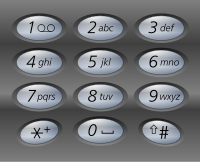## Letter Combinations of A Phone Number

06/25/2016 Backtracking String

## Question

Given a digit string, return all possible letter combinations that the number could represent.

A mapping of digit to letters (just like on the telephone buttons) is given below.Input:Digit string "23"
Output: ["ad", "ae", "af", "bd", "be", "bf", "cd", "ce", "cf"].


Note: Although the above answer is in lexicographical order, your answer could be in any order you want.

## Solution

Result: Accepted Time: 0ms

Here should be some explanations.

class Solution {
public:
const char *str = {" ","","abc","def","ghi","jkl","mno","pqrs","tuv","wxyz",};
const int  lens = {  0, 0,    3,    3,    3,    3,    3,     4,    3,    4,};
vector<string> letterCombinations(string digits) {
const int len = digits.length();
char tmp={0};
vector<string> ans;
if(!len)
return ans;
int enm = 0,rest = 0,i,t;
while(!rest)
{
for(i = 0,t,rest = enm++; i < len; i++,rest /= lens[t])/// rest /= lens[t]  and rest%lens[t] is the magic !!!!!!!
{
t = digits[i]-'0';
tmp[i] = str[t][rest%lens[t]];/// Can you figure this out ?
}
if(!rest)   ans.push_back(tmp);
}
return ans;
}
};


Complexity Analytics

• Time Complexity: $O(3^n)$
• Space Complexity: $O(n*3^n)$